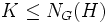# Weakly normal implies weakly closed in intermediate nilpotent

## Statement

### Statement with symbols

Suppose$H \le K \le G$ are groups such that:

•$H$ is a Weakly normal subgroup (?) of$G$.
•$K$ is a Nilpotent group (?).

Then,$H$ is a Weakly closed subgroup (?) of$K$.

## Definitions used

For these definitions,$H^g = g^{-1}Hg$ denotes the conjugate subgroup by$g \in G$. (This is the right-action convention; however, adopting a left-action convention does not alter any of the proof details).

### Paranormal subgroup

Further information: Weakly normal subgroup

A subgroup$H$ of a group$G$ is termed paranormal in$G$ if for any$g \in G$,$H^g \le N_G(H)$ implies$H^g \le H$. In other words, it is weakly closed in its normalizer.

### Weakly closed subgroup

Further information: Weakly closed subgroup

Suppose$H \le K \le G$ are groups. We say$H$ is weakly closed in$K$ with respect to$G$ if, for any$g \in G$ such that$H^g \le K$, we have$H^g \le H$.

## Proof

Given:$H \le K \le G$ with$H$ a paranormal subgroup of$G$ and$K$ a nilpotent group.

To prove: For any$g \in G$ such that$H^g \le K$, we have$H^g \le H$.

Proof: By fact (2),$H$ is a subnormal subgroup of$K$. By fact (1),$H$ is therefore normal in$K$. Thus,$K \le N_G(H)$.

Since$H$ is weakly closed in$N_G(H)$ by definition, whenever$H^g \le N_G(H)$, we get$H^g \le H$. In particular, whenever$H^g \le K$, we get$H^g \le H$, so$H$ is weakly closed in$K$.